### Home > PC > Chapter 4 > Lesson 4.1.4 > Problem4-61

4-61.
1. Solve the following equations. Homework Help ✎

1. 50x2/3 = 100

2. log3(x + 1) + log3(x) = log3 12

3. 50 (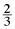)x = 100

4. log3(x + 1) − log3(x) = log3 12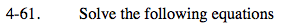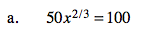Divide both side by 50 first.
Then undo the powers on both sides.

(x2/3)3/2 = 23/2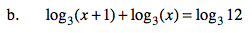Combine the terms on the left side into one log.

log3((x + 1)(x)) = log312
(x + 1)(x) = 12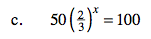Divide by 50 first.
Then take the log of both sides, bringing the x down in front.
Solve for x.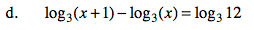This problem is similar to part (b).